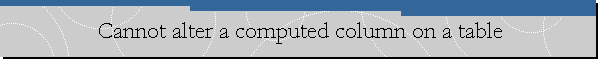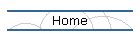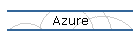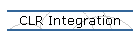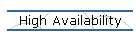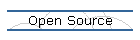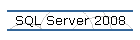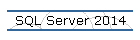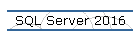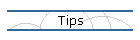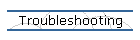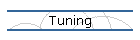## Cannot alter a computed column on a table.

Applies to: Microsoft SQL Server 2008 or later.

Problem Description.

I created a table with a computed column as shown below:

USE Morillo

CREATE TABLE [dbo].[OrderDetails](
[Price] [numeric](8,3) NOT NULL,
[SubTotal] AS [Price] * [Quantity],
[Quantity] [numeric](8,3) NOT NULL,
[Discount] [numeric](8,3) NOT NULL)

Then I decided I want to modify (alter) the computed column to include the Discount column as shown below:

ALTER TABLE dbo.[OrderDetails]
ALTER COLUMN [SubTotal] AS ([Price] * [Quantity] * [Discount])

However, when I ran the ALTER COLUMN the following error appeared:

Msg 156, Level 15, State 1, Line 2
Incorrect syntax near the keyword 'AS'.

Cause.

A computed column cannot be modified using the ALTER COLUMN argument of an ALTER TABLE statement. This is described on the "Arguments" section of this MSDN article.

Solution.

A workaround is to drop the column and recreate it.

ALTER TABLE dbo.[OrderDetails]
DROP COLUMN [SubTotal]

ALTER TABLE dbo.[OrderDetails]
ADD [SubTotal] AS ([Price] * [Quantity] * [Discount])